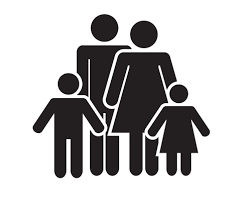# Daughter's 6484

Jana is four years old. Her father is six times more aged. In how many years will a father's age be three times his daughter's age?

r =  6

### Step-by-step explanation:

3·(4+r) = r+6·4

2r = 12

r = 12/2 = 6

r = 6

Our simple equation calculator calculates it.Did you find an error or inaccuracy? Feel free to write us. Thank you!

Tips for related online calculators
Do you have a linear equation or system of equations and looking for its solution? Or do you have a quadratic equation?
Do you want to convert time units like minutes to seconds?